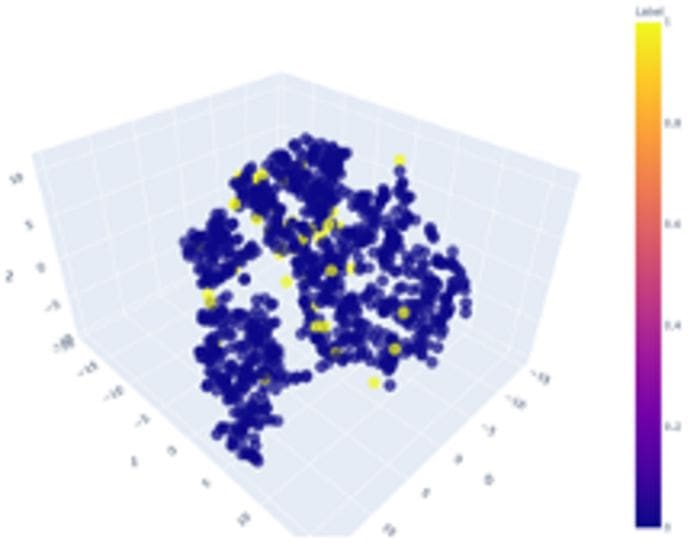# Automated Anomaly Detection Using PyCaret

Learn to automate anomaly detection using the open source machine learning library PyCaret.

By Ekta Sharma, Data Science EnthusiastPhoto Credit — Unsplash

PyCaret is an open-source library that provides a variety of machine learning functions through various modules one of which is anomaly detection.

PyCaret’s anomaly detection module is an unsupervised machine learning module that is used for identifying extreme values present in the data that can sometimes indicate suspicious activity or an abnormal instance.

PyCaret’s anomaly detection module provides twelve different anomaly detection techniques to choose from depending on the problem you are working on. It also lets us perform feature engineering tasks through a function called “setup” by using various parameter values passed to this function.

In this article, we are going to apply three of the anomaly detection techniques provided by PyCaret on one of the datasets provided by PyCaret itself. The three techniques covered in this article are — Isolation Forest, K Nearest Neighbors, and Clustering.

Before we implement any of these techniques, let’s take a look at the steps that we will need to follow in a specific order to identify anomalies in the data by using the following functions. These steps are common to all techniques provided by PyCaret for anomaly detection.

• get_data() — This function is used to access the PyCaret dataset. This is an optional step.
• setup() — This function initializes the environment and performs the preprocessing tasks needed before anomaly detection. The only required parameter that it takes is a Dataframe in the “data” parameter but below is an example of various preprocessing tasks that can be achieved through the setup function.
``setup(data, categorical_features = None, categorical_imputation = ‘constant’, ordinal_features = None, high_cardinality_features = None, numeric_features = None, numeric_imputation = ‘mean’, date_features = None, ignore_features = None, normalize = False, normalize_method = ‘zscore’, transformation = False, transformation_method = ‘yeo-johnson’, handle_unknown_categorical = True, unknown_categorical_method = ‘least_frequent’, pca = False, pca_method = ‘linear’, pca_components = None, ignore_low_variance = False, combine_rare_levels = False, rare_level_threshold = 0.10, bin_numeric_features = None, remove_multicollinearity = False, multicollinearity_threshold = 0.9, group_features = None, group_names = None, supervised = False, supervised_target = None, session_id = None, profile = False, verbose=True)``

• create_model() — This function creates the model and trains it on the dataset passed as the parameter during the setup stage. Hence, this function requires setup() function to be called before it is used.
``````df = pd.read_csv(path_to_csv) # to access your own dataset
or
df = get_data(“anomaly”) # to access PyCaret’s anomaly datasetsetup_data = setup(data=df)
sample_model = create_model(“iforest”)``````

• plot_model() — This function takes the trained model created during create_model() function and plots the data passed during the setup() function. Hence, this method requires both setup() and create_model() function to be called before it is called. The returned plot cleary shows anomaly data in a different color.
``plot_model(sample_model)``

• predict_model() — This function takes the trained model and uses it to make predictions on the new data. The new data must be in the form of a Pandas Dataframe. The output of this function is a Dataframe with predictions called “Label” and the associated decision Score.

Label = 0 means normal data or inlier

Label = 1 means an anomaly or outlier

Now, when we have a basic understanding of how PyCaret Anomaly Detection Functions work, let’s dive into the actual implementation.

``````# Importing PyCaret dependencies.
from pycaret.datasets import get_data
anomaly = get_data(“anomaly”)# Importing anomaly detection module.
from pycaret.anomaly import *# Initializing the setup function used for pre-processing.
setup_anomaly_data = setup(anomaly)``````

### Isolation Forest Implementation

``````# Instantiating Isolation Forest model.
iforest = create_model(“iforest”)# Plotting the data using Isolation Forest model.
plot_model(iforest)# Generating the predictions using Isolation Forest trained model.
iforest_predictions = predict_model(iforest, data = anomaly)
print(iforest_predictions)# Checking anomaly rows. Label = 1 is the anomaly data.
iforest_anomaly_rows = iforest_predictions[iforest_predictions[“Label”] == 1]
print(iforest_anomaly_rows.head())# Checking the number of anomaly rows returned by Isolaton Forest.
print(iforest_anomaly_rows.shape) # returned 50 rows``````Top 5 Anomaly Rows (Label 1)Anomaly Plots created using Isolation Forest (Anomaly highlighted in Yellow color)Isolation Forest Based Anomaly Plot

### K Nearest Neighbors (KNN) Implementation

``````# Instantiating KNN model.
knn = create_model(“knn”)# Plotting the data using KNN model.
plot_model(knn)# Generating the predictions using KNN trained model.
knn_predictions = predict_model(knn, data = anomaly)
print(knn_predictions)# Checking KNN anomaly rows. Predictions with Label = 1 are anomalies.
knn_anomaly_rows = knn_predictions[knn_predictions[“Label”] == 1]
knn_anomaly_rows.head()# Checking the number of anomaly rows returned by KNN model.
knn_anomaly_rows.shape # returned 46 rows``````Top 5 Anomaly Rows (Label 1)Anomaly Plot created using K Nearest Neighbors (Anomaly highlighted in Yellow color)KNN Based Anomaly Plot

### Clustering Implementation

``````# Instantiating Cluster model.
cluster = create_model(“cluster”)# Plotting the data using Cluster model.
plot_model(cluster)# Generating the predictions using Cluster trained model.
cluster_predictions = predict_model(cluster, data = anomaly)
print(cluster_predictions)# Checking cluster anomaly rows. Predictions with Label = 1 are anomalies.
cluster_anomaly_rows = cluster_predictions[cluster_predictions[“Label”] == 1]
print(cluster_anomaly_rows.head())# Checking the number of anomaly rows returned by Cluster model.
cluster_anomaly_rows.shape # returned 50 rows``````Top 5 Anomaly Rows (Label 1)Anomaly Plot created using Clustering (Anomaly highlighted in Yellow color)Clustering Based Anomaly Plot

References

Bio: Ekta Sharma is a Data Science Enthusiast.

Original. Reposted with permission.

Related:

By subscribing you accept KDnuggets Privacy Policy

By subscribing you accept KDnuggets Privacy Policy

By subscribing you accept KDnuggets Privacy Policy